# 12-Image enhancement and filtering.ppt

23 de Apr de 2023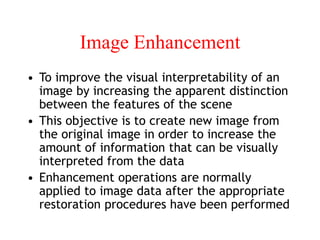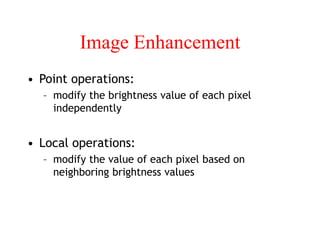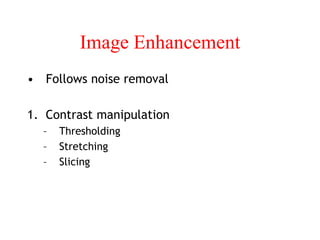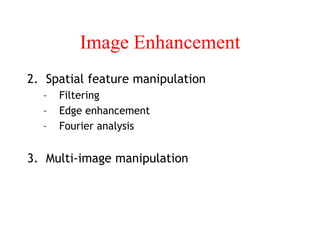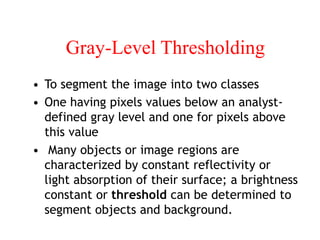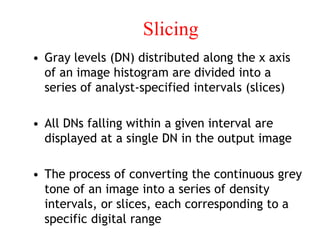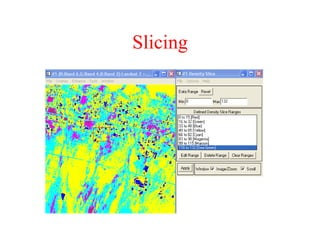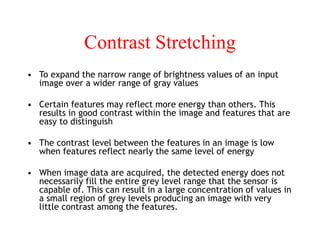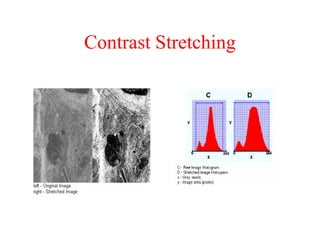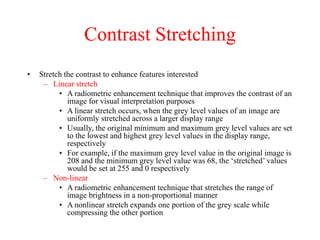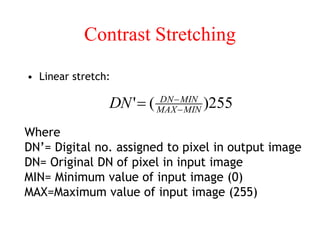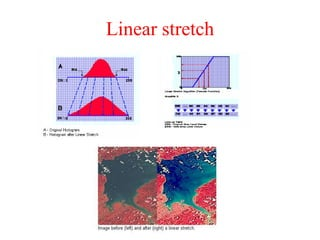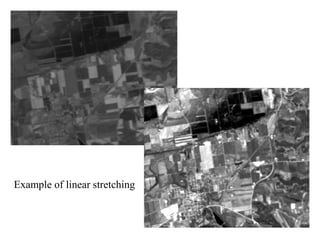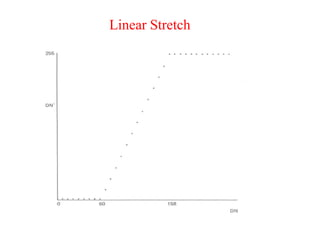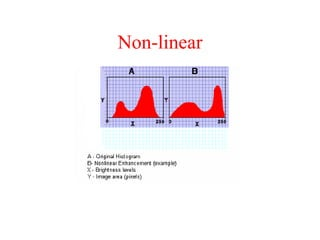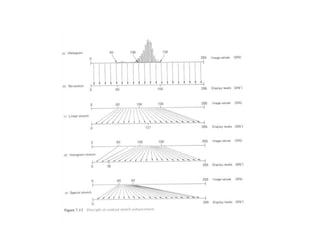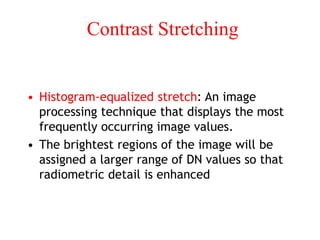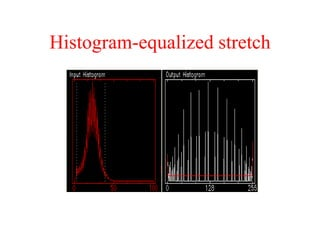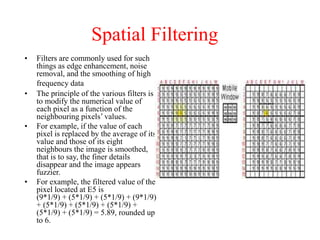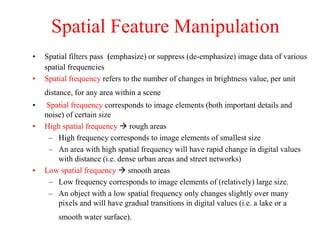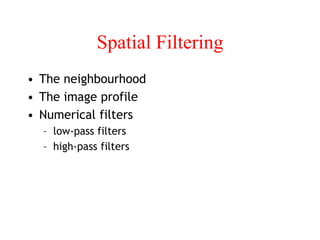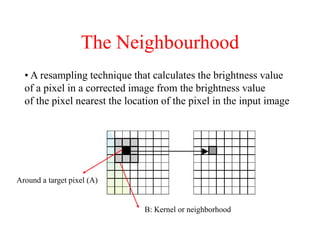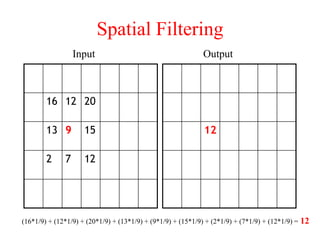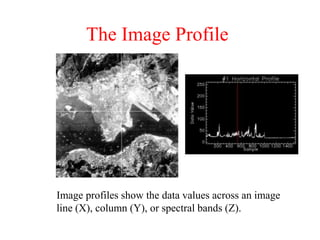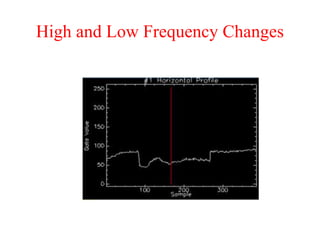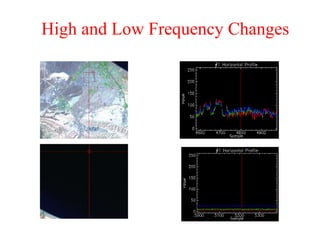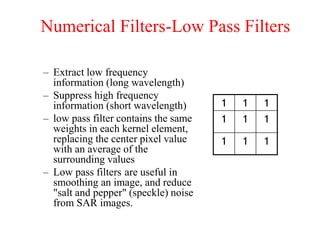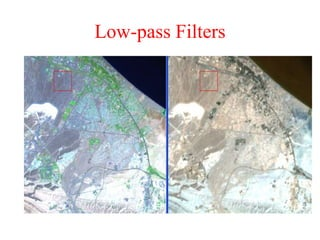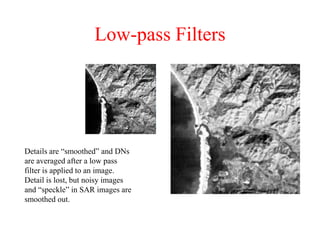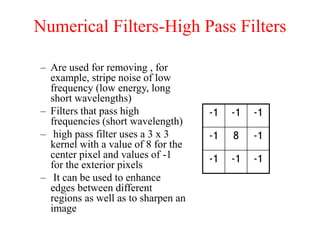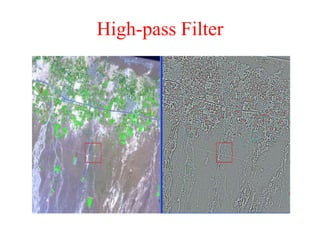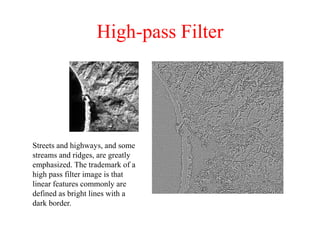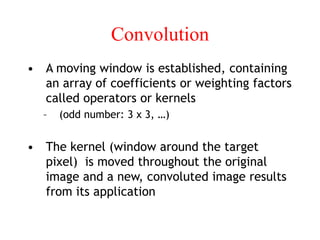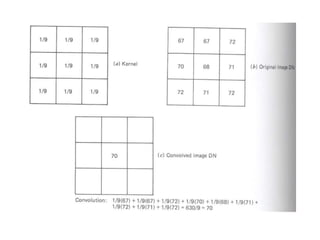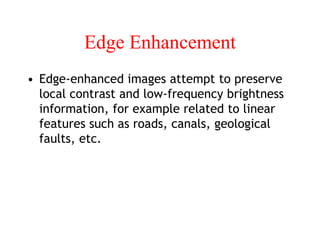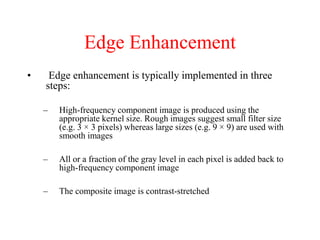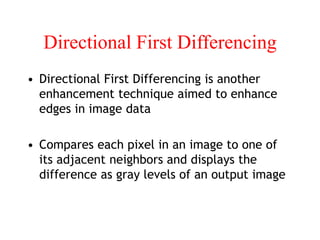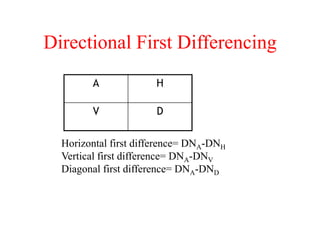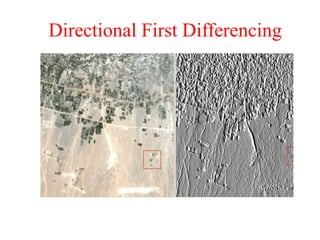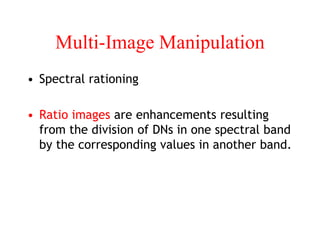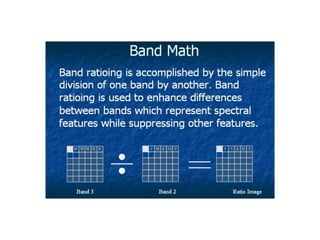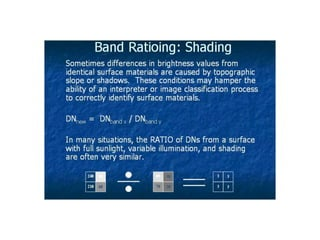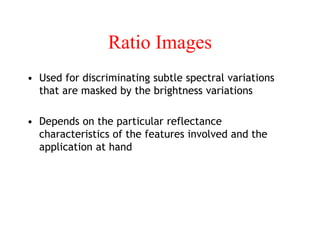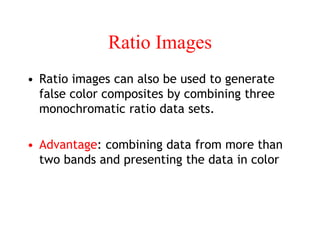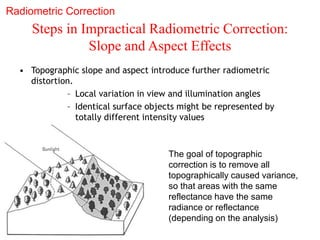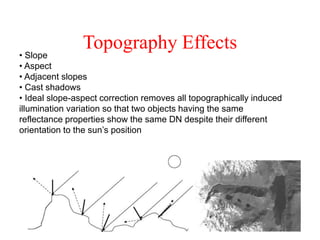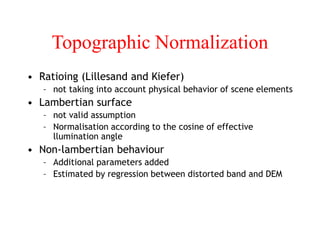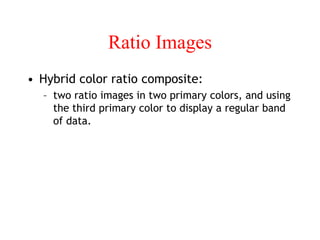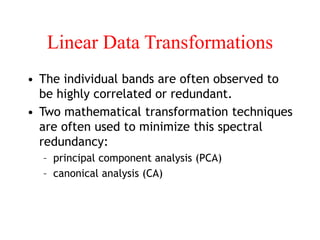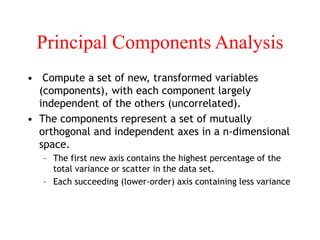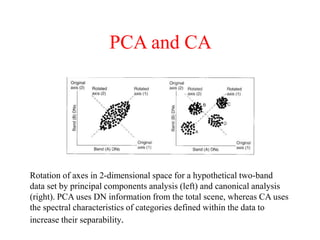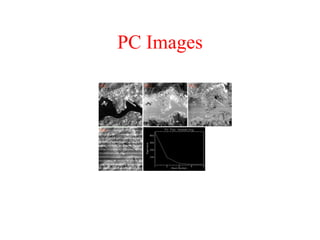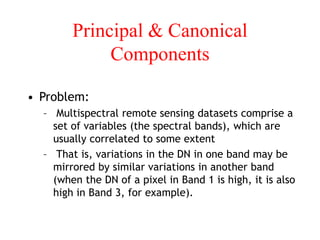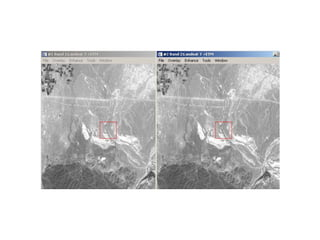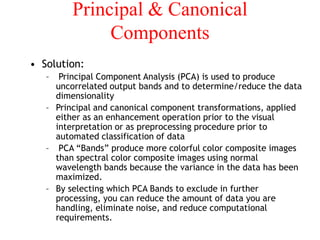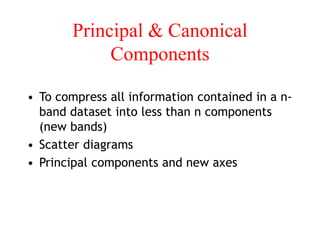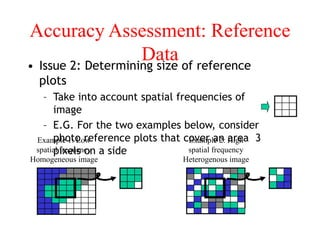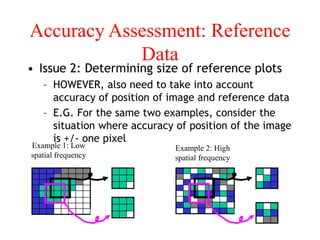1 de 60

### 12-Image enhancement and filtering.ppt

• 1. Image Enhancement • To improve the visual interpretability of an image by increasing the apparent distinction between the features of the scene • This objective is to create new image from the original image in order to increase the amount of information that can be visually interpreted from the data • Enhancement operations are normally applied to image data after the appropriate restoration procedures have been performed
• 2. Image Enhancement • Point operations: – modify the brightness value of each pixel independently • Local operations: – modify the value of each pixel based on neighboring brightness values
• 3. Image Enhancement • Follows noise removal 1. Contrast manipulation – Thresholding – Stretching – Slicing
• 4. Image Enhancement 2. Spatial feature manipulation – Filtering – Edge enhancement – Fourier analysis 3. Multi-image manipulation
• 5. Gray-Level Thresholding • To segment the image into two classes • One having pixels values below an analyst- defined gray level and one for pixels above this value • Many objects or image regions are characterized by constant reflectivity or light absorption of their surface; a brightness constant or threshold can be determined to segment objects and background.
• 6. Slicing • Gray levels (DN) distributed along the x axis of an image histogram are divided into a series of analyst-specified intervals (slices) • All DNs falling within a given interval are displayed at a single DN in the output image • The process of converting the continuous grey tone of an image into a series of density intervals, or slices, each corresponding to a specific digital range
• 8. Contrast Stretching • To expand the narrow range of brightness values of an input image over a wider range of gray values • Certain features may reflect more energy than others. This results in good contrast within the image and features that are easy to distinguish • The contrast level between the features in an image is low when features reflect nearly the same level of energy • When image data are acquired, the detected energy does not necessarily fill the entire grey level range that the sensor is capable of. This can result in a large concentration of values in a small region of grey levels producing an image with very little contrast among the features.
• 10. Contrast Stretching • Stretch the contrast to enhance features interested – Linear stretch • A radiometric enhancement technique that improves the contrast of an image for visual interpretation purposes • A linear stretch occurs, when the grey level values of an image are uniformly stretched across a larger display range • Usually, the original minimum and maximum grey level values are set to the lowest and highest grey level values in the display range, respectively • For example, if the maximum grey level value in the original image is 208 and the minimum grey level value was 68, the ‘stretched’ values would be set at 255 and 0 respectively – Non-linear • A radiometric enhancement technique that stretches the range of image brightness in a non-proportional manner • A nonlinear stretch expands one portion of the grey scale while compressing the other portion
• 11. Contrast Stretching • Linear stretch: Where DN’= Digital no. assigned to pixel in output image DN= Original DN of pixel in input image MIN= Minimum value of input image (0) MAX=Maximum value of input image (255)  DN' ( DNMIN MAXMIN )255
• 13. Example of linear stretching
• 17. Contrast Stretching • Histogram-equalized stretch: An image processing technique that displays the most frequently occurring image values. • The brightest regions of the image will be assigned a larger range of DN values so that radiometric detail is enhanced
• 19. Spatial Filtering • Filters are commonly used for such things as edge enhancement, noise removal, and the smoothing of high frequency data • The principle of the various filters is to modify the numerical value of each pixel as a function of the neighbouring pixels’ values. • For example, if the value of each pixel is replaced by the average of its value and those of its eight neighbours the image is smoothed, that is to say, the finer details disappear and the image appears fuzzier. • For example, the filtered value of the pixel located at E5 is (9*1/9) + (5*1/9) + (5*1/9) + (9*1/9) + (5*1/9) + (5*1/9) + (5*1/9) + (5*1/9) + (5*1/9) = 5.89, rounded up to 6.
• 20. Spatial Feature Manipulation • Spatial filters pass (emphasize) or suppress (de-emphasize) image data of various spatial frequencies • Spatial frequency refers to the number of changes in brightness value, per unit distance, for any area within a scene • Spatial frequency corresponds to image elements (both important details and noise) of certain size • High spatial frequency  rough areas – High frequency corresponds to image elements of smallest size – An area with high spatial frequency will have rapid change in digital values with distance (i.e. dense urban areas and street networks) • Low spatial frequency  smooth areas – Low frequency corresponds to image elements of (relatively) large size. – An object with a low spatial frequency only changes slightly over many pixels and will have gradual transitions in digital values (i.e. a lake or a smooth water surface).
• 21. Spatial Filtering • The neighbourhood • The image profile • Numerical filters – low-pass filters – high-pass filters
• 22. The Neighbourhood • A resampling technique that calculates the brightness value of a pixel in a corrected image from the brightness value of the pixel nearest the location of the pixel in the input image B: Kernel or neighborhood Around a target pixel (A)
• 23. Spatial Filtering 16 12 20 13 9 15 2 7 12 12 Input Output (16*1/9) + (12*1/9) + (20*1/9) + (13*1/9) + (9*1/9) + (15*1/9) + (2*1/9) + (7*1/9) + (12*1/9) = 12
• 24. The Image Profile Image profiles show the data values across an image line (X), column (Y), or spectral bands (Z).
• 25. High and Low Frequency Changes
• 26. High and Low Frequency Changes
• 27. Numerical Filters-Low Pass Filters – Extract low frequency information (long wavelength) – Suppress high frequency information (short wavelength) – low pass filter contains the same weights in each kernel element, replacing the center pixel value with an average of the surrounding values – Low pass filters are useful in smoothing an image, and reduce "salt and pepper" (speckle) noise from SAR images. 1 1 1 1 1 1 1 1 1
• 29. Low-pass Filters Details are “smoothed” and DNs are averaged after a low pass filter is applied to an image. Detail is lost, but noisy images and “speckle” in SAR images are smoothed out.
• 30. Numerical Filters-High Pass Filters – Are used for removing , for example, stripe noise of low frequency (low energy, long short wavelengths) – Filters that pass high frequencies (short wavelength) – high pass filter uses a 3 x 3 kernel with a value of 8 for the center pixel and values of -1 for the exterior pixels – It can be used to enhance edges between different regions as well as to sharpen an image -1 -1 -1 -1 8 -1 -1 -1 -1
• 32. High-pass Filter Streets and highways, and some streams and ridges, are greatly emphasized. The trademark of a high pass filter image is that linear features commonly are defined as bright lines with a dark border.
• 33. Convolution • A moving window is established, containing an array of coefficients or weighting factors called operators or kernels – (odd number: 3 x 3, …) • The kernel (window around the target pixel) is moved throughout the original image and a new, convoluted image results from its application
• 35. Edge Enhancement • Edge-enhanced images attempt to preserve local contrast and low-frequency brightness information, for example related to linear features such as roads, canals, geological faults, etc.
• 36. Edge Enhancement • Edge enhancement is typically implemented in three steps: – High-frequency component image is produced using the appropriate kernel size. Rough images suggest small filter size (e.g. 3 × 3 pixels) whereas large sizes (e.g. 9 × 9) are used with smooth images – All or a fraction of the gray level in each pixel is added back to high-frequency component image – The composite image is contrast-stretched
• 37. Directional First Differencing • Directional First Differencing is another enhancement technique aimed to enhance edges in image data • Compares each pixel in an image to one of its adjacent neighbors and displays the difference as gray levels of an output image
• 38. Directional First Differencing A H V D Horizontal first difference= DNA-DNH Vertical first difference= DNA-DNV Diagonal first difference= DNA-DND
• 40. Multi-Image Manipulation • Spectral rationing • Ratio images are enhancements resulting from the division of DNs in one spectral band by the corresponding values in another band.
• 43. Ratio Images • Used for discriminating subtle spectral variations that are masked by the brightness variations • Depends on the particular reflectance characteristics of the features involved and the application at hand
• 44. Ratio Images • Ratio images can also be used to generate false color composites by combining three monochromatic ratio data sets. • Advantage: combining data from more than two bands and presenting the data in color
• 45. Steps in Impractical Radiometric Correction: Slope and Aspect Effects • Topographic slope and aspect introduce further radiometric distortion. – Local variation in view and illumination angles – Identical surface objects might be represented by totally different intensity values The goal of topographic correction is to remove all topographically caused variance, so that areas with the same reflectance have the same radiance or reflectance (depending on the analysis) Radiometric Correction
• 46. Topography Effects • Slope • Aspect • Adjacent slopes • Cast shadows • Ideal slope-aspect correction removes all topographically induced illumination variation so that two objects having the same reflectance properties show the same DN despite their different orientation to the sun’s position
• 47. Topographic Normalization • Ratioing (Lillesand and Kiefer) – not taking into account physical behavior of scene elements • Lambertian surface – not valid assumption – Normalisation according to the cosine of effective llumination angle • Non-lambertian behaviour – Additional parameters added – Estimated by regression between distorted band and DEM
• 48. Ratio Images • Hybrid color ratio composite: – two ratio images in two primary colors, and using the third primary color to display a regular band of data.
• 49. Linear Data Transformations • The individual bands are often observed to be highly correlated or redundant. • Two mathematical transformation techniques are often used to minimize this spectral redundancy: – principal component analysis (PCA) – canonical analysis (CA)
• 50. Principal Components Analysis • Compute a set of new, transformed variables (components), with each component largely independent of the others (uncorrelated). • The components represent a set of mutually orthogonal and independent axes in a n-dimensional space. – The first new axis contains the highest percentage of the total variance or scatter in the data set. – Each succeeding (lower-order) axis containing less variance
• 51. PCA and CA Rotation of axes in 2-dimensional space for a hypothetical two-band data set by principal components analysis (left) and canonical analysis (right). PCA uses DN information from the total scene, whereas CA uses the spectral characteristics of categories defined within the data to increase their separability.
• 53. Principal & Canonical Components • Problem: – Multispectral remote sensing datasets comprise a set of variables (the spectral bands), which are usually correlated to some extent – That is, variations in the DN in one band may be mirrored by similar variations in another band (when the DN of a pixel in Band 1 is high, it is also high in Band 3, for example).
• 55. Principal & Canonical Components • Solution: – Principal Component Analysis (PCA) is used to produce uncorrelated output bands and to determine/reduce the data dimensionality – Principal and canonical component transformations, applied either as an enhancement operation prior to the visual interpretation or as preprocessing procedure prior to automated classification of data – PCA “Bands” produce more colorful color composite images than spectral color composite images using normal wavelength bands because the variance in the data has been maximized. – By selecting which PCA Bands to exclude in further processing, you can reduce the amount of data you are handling, eliminate noise, and reduce computational requirements.
• 56. Principal & Canonical Components • To compress all information contained in a n- band dataset into less than n components (new bands) • Scatter diagrams • Principal components and new axes
• 57. Principal & Canonical Components • To compress all information contained in a n- band dataset into less than n components (new bands) • Scatter diagrams • Principal components and new axes
• 58. Principal & Canonical Components • To compress all information contained in a n- band dataset into less than n components (new bands) • Scatter diagrams • Principal components and new axes
• 59. Accuracy Assessment: Reference Data • Issue 2: Determining size of reference plots – Take into account spatial frequencies of image – E.G. For the two examples below, consider photo reference plots that cover an area 3 pixels on a side Example 1: Low spatial frequency Homogeneous image Example 2: High spatial frequency Heterogenous image
• 60. Accuracy Assessment: Reference Data • Issue 2: Determining size of reference plots – HOWEVER, also need to take into account accuracy of position of image and reference data – E.G. For the same two examples, consider the situation where accuracy of position of the image is +/- one pixel Example 1: Low spatial frequency Example 2: High spatial frequency